# # 块级作用域：var缺陷以及为什么要引入let和const

## # 作用域（scope）

• 全局作用域中的对象在代码中的任何地方都能访问，其生命周期伴随着页面的生命周期。
• 函数作用域就是在函数内部定义的变量或者函数，并且定义的变量或者函数只能在函数内部被访问。函数执行结束之后，函数内部定义的变量会被销毁。

``````//if块
if(1){}

//while块
while(1){}

//函数块
function foo(){

//for循环块
for(let i = 0; i<100; i++){}

//单独一个块
{}
``````

``````char* myname = "极客时间";
void showName() {
printf("%s \n",myname);
if(0){
char* myname = "极客邦";
}
}

int main(){
showName();
return 0;
}
``````

## # 变量提升所带来的问题

### # 1. 变量容易在不被察觉的情况下被覆盖掉

``````var myname = "极客时间"
function showName(){
console.log(myname);
if(0){
var myname = "极客邦"
}
console.log(myname);
}
showName()
``````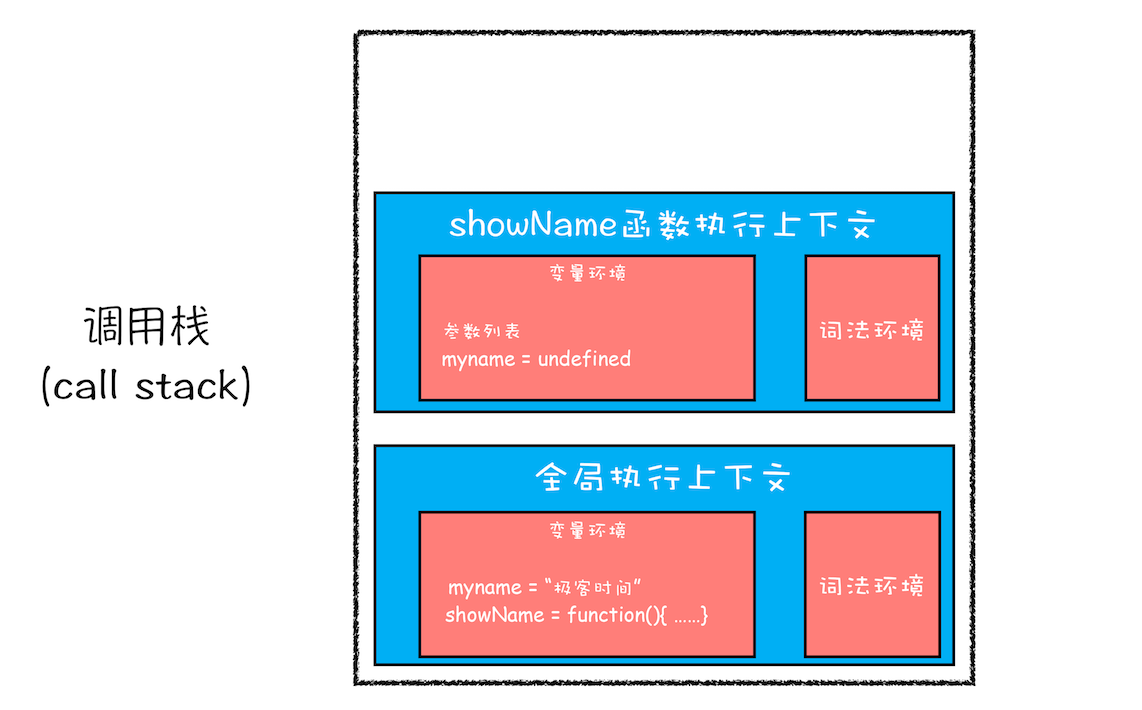showName函数的执行上下文创建后，JavaScript引擎便开始执行showName函数内部的代码了。首先执行的是：

``````console.log(myname);
``````

### # 2. 本应销毁的变量没有被销毁

``````function foo(){
for (var i = 0; i < 7; i++) {
}
console.log(i);
}
foo()
``````

## # ES6是如何解决变量提升带来的缺陷

``````let x = 5
const y = 6
x = 7
y = 9 //报错，const声明的变量不可以修改
``````

``````function varTest() {
var x = 1;
if (true) {
var x = 2;  // 同样的变量!
console.log(x);  // 2
}
console.log(x);  // 2
}
``````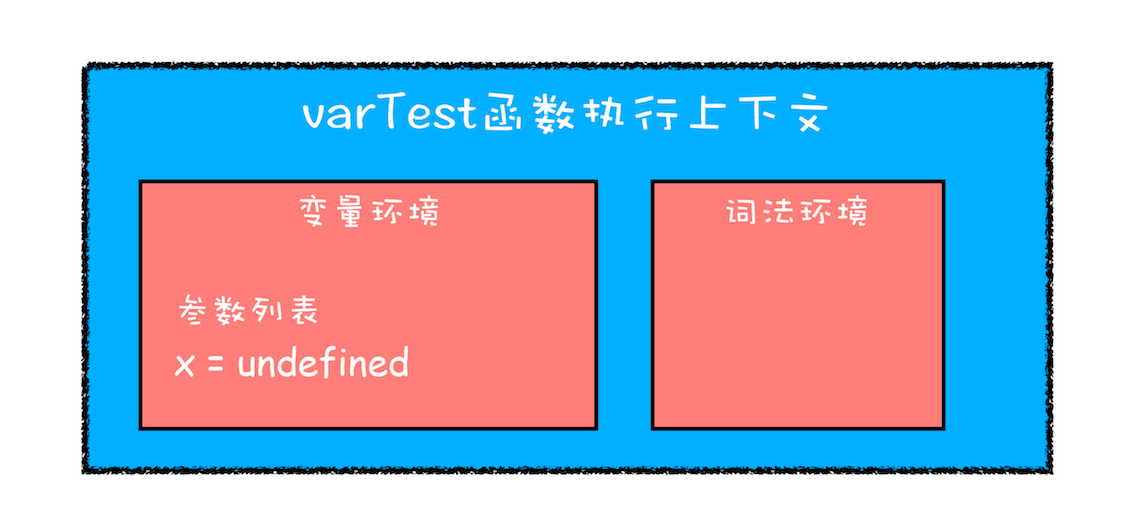``````function letTest() {
let x = 1;
if (true) {
let x = 2;  // 不同的变量
console.log(x);  // 2
}
console.log(x);  // 1
}
``````

## # JavaScript是如何支持块级作用域的

``````function foo(){
var a = 1
let b = 2
{
let b = 3
var c = 4
let d = 5
console.log(a)
console.log(b)
}
console.log(b)
console.log(c)
console.log(d)
}
foo()
``````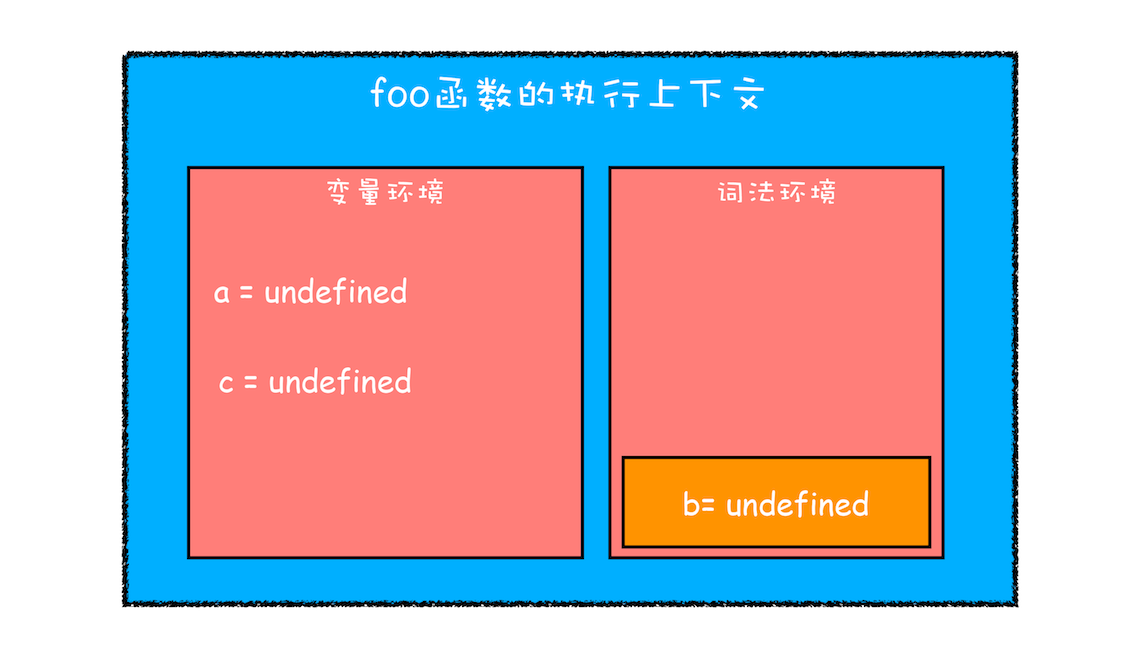• 函数内部通过var声明的变量，在编译阶段全都被存放到变量环境里面了。
• 通过let声明的变量，在编译阶段会被存放到词法环境（Lexical Environment）中。
• 在函数的作用域内部，通过let声明的变量并没有被存放到词法环境中。
• 接下来，第二步继续执行代码，当执行到代码块里面时，变量环境中a的值已经被设置成了1，词法环境中b的值已经被设置成了2，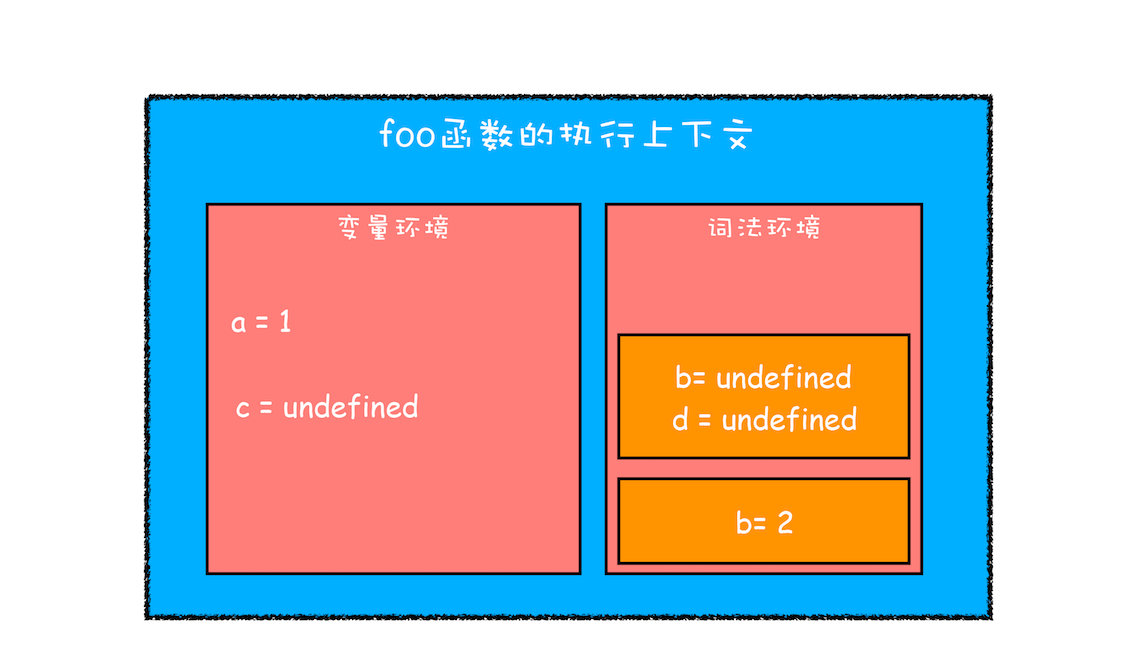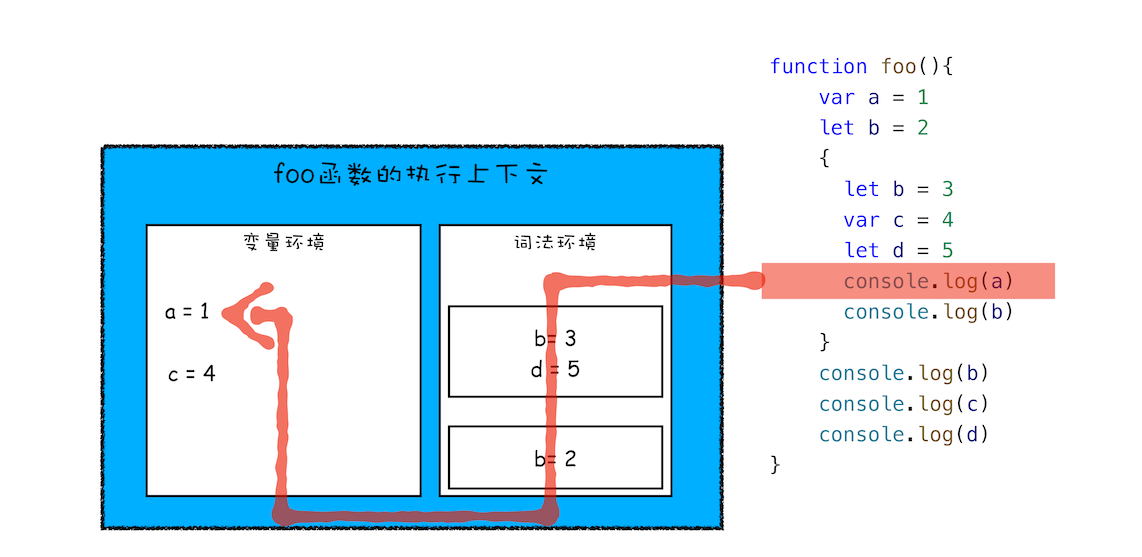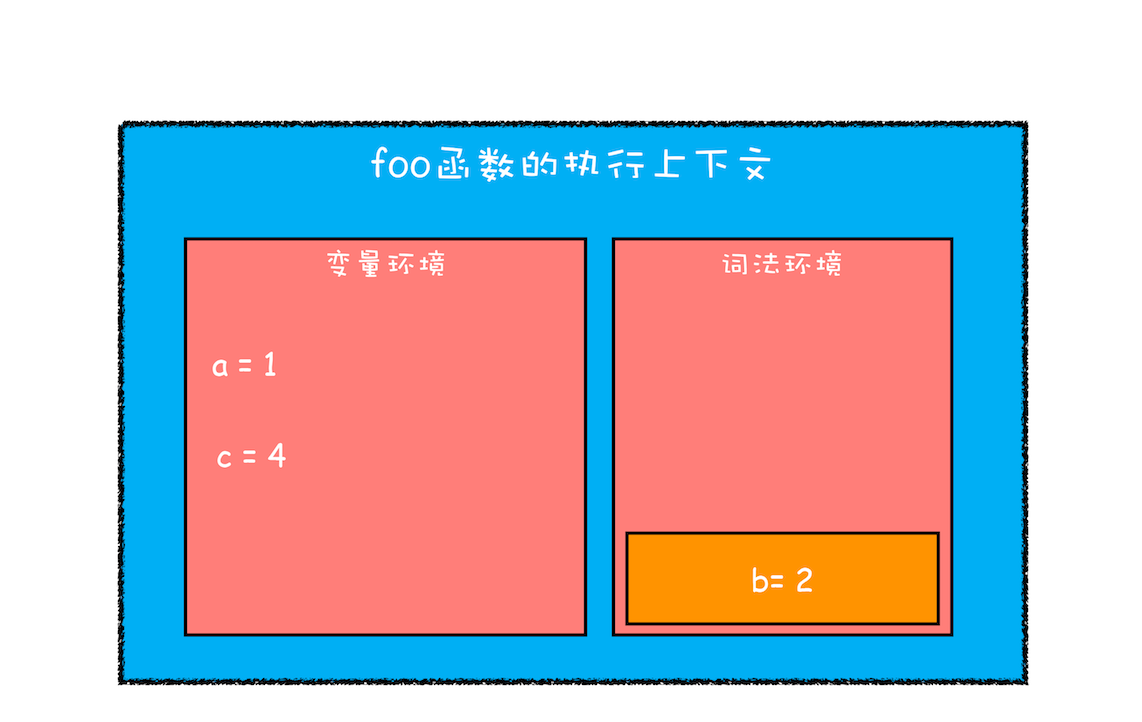## # 思考时间

``````let myname= '极客时间'
{
console.log(myname)
let myname= '极客邦'
}
``````

1. 在块级作用域中，从`开始到let myname= '极客邦'`代码之间会形成一个暂时性死区，如果中间去访问变量`myname`，会报初始化之前不能访问`myname`的错误。`Uncaught ReferenceError`

2. 另外上面的一个foo函数也会报d没有定义，d在块级作用域中声明，在外面是访问不到的

``````function foo(){
var a = 1
let b = 2
{
let b = 3
var c = 4
let d = 5
console.log(a)
console.log(b)
}
console.log(b)
console.log(c)
console.log(d)
}
foo()
``````